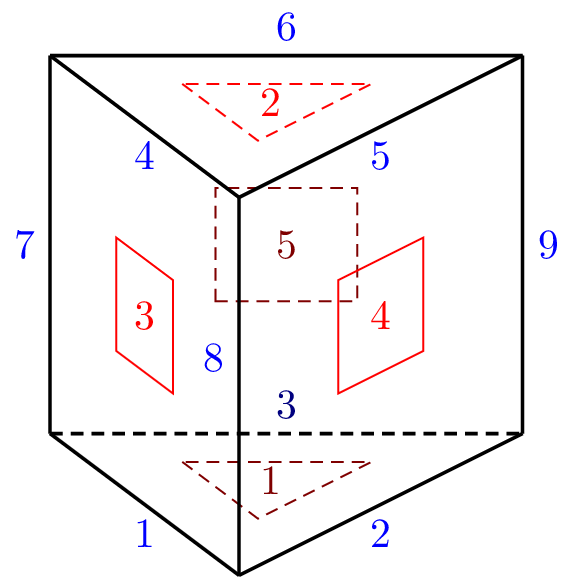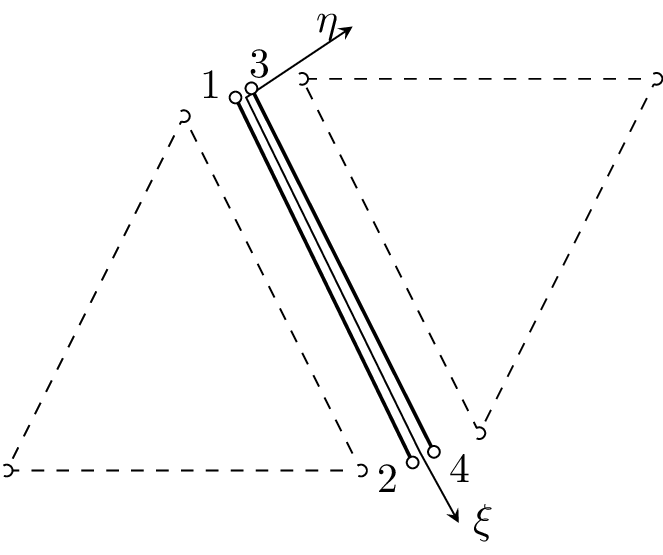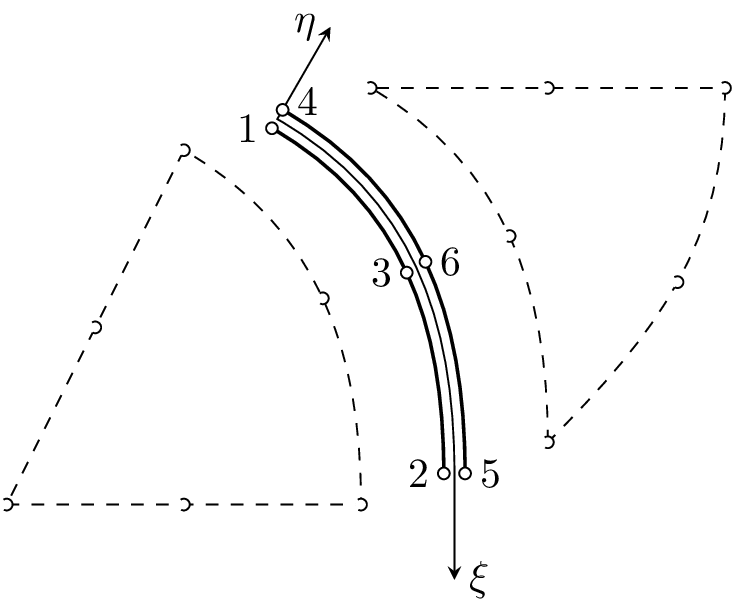#### 2.9 Interface elements

Interface elements represents an interaction between points, edges or surfaces. They are used to model debonding between surfaces or more general fracture processes through the use of cohesive zone (cz) models. They can also be used to model contact between elements. Specific interface material models needs to be used - consult the matlibmanual for supported models.

Ordering convention: All inerface elements have plus-side and a minus-side and all nodes should first be specified for the minus-side and then the plus-side. The normal to the interface is defined to point from the minus-side to the-plus side. Direction of normal vector on an element specifies normal stress/cohesion across the element. It is assumed that normal jump, normal traction are at the first position of corresponding vectors. Stiffness matrix in local coordinates has always on position 1,1 normal stiffness and then shear stiffness.

##### 2.9.1 IntElPoint, Interface1d elements

Implementation of one dimensional (slip) interface element. This element connects two separate nodes and the interaction is governed by a one-dimensional slip law. This law determines the force acting between the nodes as a function on their relative displacement in the slip direction. The element can be used in 1D, 2D, and 3D (default) and its features are summarized in Table 39.

 Keyword IntElPoint, Interface1d (deprecated) Description One dimensional (slip) interface element Specific parameters [refnode #(in)] [normal #(ra)] Parameters refnode: determines the reference node, which is used to specify a reference direction (the direction vector is obtained by subtracting the coordinates of the first node from the reference node). normal: The reference direction can be directly specified by the optional parameter normal. Although both refnode and normal are optional, at least one of them must be specified. Unknowns One, two, or three DOFs (u-displacement, v-displacement, w-displacement) are required in each node, according to element mode (determined from domain type). Approximation - Integration - Features - CS properties - Loads - Nlgeo 0 Status Reliable Note Element requires material model with _1dInterface support.

Table 39: IntElPoint element summary

##### 2.9.2 IntElLine1, Interface2dlin elements

Implementation of a two dimensional line element with a linear approximation of the displacement jump. The element can be used to tie together two element edges and is defined by four nodes - two on each edge. Note that, the nodes along the interface are doubled, each couple with identical coordinates. Nodes on the negative side are numbered first, followed by nodes on the positive part. Requires material model with _2dInterface support. The element features are summarized in Table ??.Figure 18: Interface2dlin element with linear interpolation. Definition and node numbering convention

 Keyword IntElLine1, Interface2dlin(deprecated) Description 2D interface element with linear approximation Specific parameters [axisymmode #()] Parameters axisymmode: Flag controlling axisymmetric mode (integration over unit circumferential angle). Unknowns Two dofs (u-displacement, v-displacement) are required in each node. Approximation Linear approximation of displacements and geometry. Integration Full integration of all strain components using two point integration formula. Features - CS properties - Loads - Nlgeo 0 Status Reliable Note Element requires material model with _2dInterface support.

Table 40: IntElLine1 element summary

##### 2.9.3 IntElLine2, Interface2dquad elements

Implementation of a two dimensional interface element with quadratic approximation of displacement field. Can be used to glue together two elements with quadratic displacement approximation along the shared edge. Note, that the nodes along the interface are doubled, each couple with identical coordinates. Nodes on the negative side are numbered first, followed by nodes on the positive part. Requires material model with _2dInterface support. The element features are summarized in Table ??.Figure 19: Interface2dquad element with quadratic interpolation. Definition and node numbering convention

 Keyword IntElLine2, Interface2dquad (deprecated) Description 2D interface element with quadratic approximation Specific parameters [axisymmode #()] Parameters axisymmode: Flag controlling axisymmetric mode (integration over unit circumferential angle). Unknowns Two dofs (u-displacement, v-displacement) are required in each node. Approximation Quadratic approximation of displacements and geometry. Integration Full integration of all strain components using four point integration formula. Features - CS properties - Loads - Nlgeo 0 Status Reliable Note Element requires material model with _2dInterface support.

Table 41: IntElLine2 element summary

##### 2.9.4 IntElSurfTr1, Interface3dtrlin elements

Implementation of a three dimensional interface element with linear approximation of displacement field. Can be used to glue together two elements with linear displacement approximation along the shared triangular surface. Note, that the nodes along the interface are doubled, each couple with identical coordinates. Nodes on the negative surface are numbered first, followed by nodes on the positive part. The numbering of surface nodes on positive surface (+) determines the positive normal (right hand rule). Requires material model with _3dInterface support. The element features are summarized in Table 42.Figure 20: Interface3dtrlin element with linear interpolation. Definition and node numbering convention

 Keyword IntElSurfTr1, Interface3dtrlin (deprecated) Description 3D interface element with linear approximation Specific parameters - Unknowns Three dofs (u-displacement, v-displacement, w-displacement) are required in each node. Approximation Linear approximation of displacements and geometry. Integration Full integration of all components using one point integration formula. Features - CS properties - Loads - Nlgeo 0 Status Reliable Note Element requires material model with _3dInterface support.

Table 42: IntElSurfTr1 element summary